JMSLTM Numerical Library 7.2.0
com.imsl.math

## Class BsInterpolate

• All Implemented Interfaces:
Serializable, Cloneable

```public class BsInterpolate
extends BSpline```
Extension of the BSpline class to interpolate data points.

Given the data points x = `xData`, f = `yData`, and n the number of elements in `xData` and `yData`, the default action of `BsInterpolate` computes a cubic (order = 4) spline interpolant s to the data using a default "not-a-knot" knot sequence. Constructors are also provided that allow the order and knot sequence to be specified. This algorithm is based on the routine `SPLINT` by de Boor (1978, p. 204).

First, the `xData` vector is sorted and the result is stored in x. The elements of `yData` are permuted appropriately and stored in f, yielding the equivalent data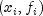for i = 0 to n-1. The following preliminary checks are performed on the data, with k = `order`. We verify that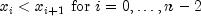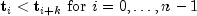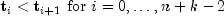The first test checks to see that the abscissas are distinct. The second and third inequalities verify that a valid knot sequence has been specified.

In order for the interpolation matrix to be nonsingular, we also checkfor i = 0 to n-1. This first inequality in the last check is necessary since the method used to generate the entries of the interpolation matrix requires that the k possibly nonzero B-splines at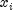,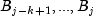where j satisfies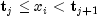be well-defined (that is,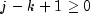).

Example, Serialized Form

• ### Fields inherited from class com.imsl.math.BSpline

`coef, knot, order`
• ### Constructor Summary

Constructors
Constructor and Description
```BsInterpolate(double[] xData, double[] yData)```
Constructs a B-spline that interpolates the given data points.
```BsInterpolate(double[] xData, double[] yData, int order)```
Constructs a B-spline that interpolates the given data points and order, using a default "not-a-knot" spline knot sequence.
```BsInterpolate(double[] xData, double[] yData, int order, double[] knot)```
Constructs a B-spline that interpolates the given data points, using the specified order and knots.

• ### Methods inherited from class com.imsl.math.BSpline

`derivative, derivative, derivative, getKnots, getSpline, integral, value, value`
• ### Methods inherited from class java.lang.Object

`clone, equals, finalize, getClass, hashCode, notify, notifyAll, toString, wait, wait, wait`
• ### Constructor Detail

• #### BsInterpolate

```public BsInterpolate(double[] xData,
double[] yData)```
Constructs a B-spline that interpolates the given data points. The computed B-spline will be order 4 (cubic) and have a default "not-a-knot" spline knot sequence.
Parameters:
`xData` - A `double` array containing the x-coordinates of the data. Values must be distinct.
`yData` - A `double` array containing the y-coordinates of the data. The arrays xData and yData must have the same length.
• #### BsInterpolate

```public BsInterpolate(double[] xData,
double[] yData,
int order)```
Constructs a B-spline that interpolates the given data points and order, using a default "not-a-knot" spline knot sequence.
Parameters:
`xData` - A `double` array containing the x-coordinates of the data. Values must be distinct.
`yData` - A `double` array containing the y-coordinates of the data.The arrays xData and yData must have the same length.
`order` - An `int` denoting the order of the B-spline.
• #### BsInterpolate

```public BsInterpolate(double[] xData,
double[] yData,
int order,
double[] knot)```
Constructs a B-spline that interpolates the given data points, using the specified order and knots.
Parameters:
`xData` - A `double` array containing the x-coordinates of the data. Values must be distinct.
`yData` - A `double` array containing the y-coordinates of the data.The arrays xData and yData must have the same length.
`order` - An `int` denoting the order of the spline.
`knot` - A `double` array containing the knot sequence for the B-spline.
JMSLTM Numerical Library 7.2.0